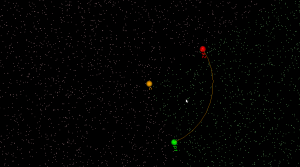## Arc predicates

I started working on arc-sites for OpenVoronoi. The first things required are an `in_region(p)` predicate and an `apex_point(p)` function. It is best to rapidly try out and visualize things in python/VTK first, before committing to slower coding and design in c++.

`in_region(p)` returns true if point `p` is inside the cone-of-influence of the arc site. Here the arc is defined by its start-point p1, end-point p2, center c, and a direction flag cw for indicating cw or ccw direction. This code will only work for arcs smaller than 180 degrees.

```def arc_in_region(p1,p2,c,cw,p): if cw: return p.is_right(c,p1) and (not p.is_right(c,p2)) else: return (not p.is_right(c,p1)) and p.is_right(c,p2)```Here randomly chosen points are shown green if they are in-region and pink if they are not.

`apex_point(p)` returns the closest point to `p` on the arc. When `p` is not in the cone-of-influence either the start- or end-point of the arc is returned. This is useful in OpenVoronoi for calculating the minimum distance from `p` to any point on the arc-site, since this is given by `(p-apex_point(p)).norm()`.

```def closer_endpoint(p1,p2,p): if (p1-p).norm() < (p2-p).norm(): return p1 else: return p2   def projection_point(p1,p2,c1,cw,p): if p==c1: return p1 else: n = (p-c1) n.normalize() return c1 + (p1-c1).norm()*n   def apex_point(p1,p2,c1,cw,p): if arc_in_region(p1,p2,c1,cw,p): return projection_point(p1,p2,c1,cw,p) else: return closer_endpoint(p1,p2,p)```Here a line from a randomly chosen point `p` to its `apex_point(p)` has been drawn. Either the start- or end-point of the arc is the closest point to out-of-region points (pink), while a radially projected point on the arc-site is closest to in-region points (green).

The next thing required are working edge-parametrizations for the new type of voronoi-edges that will occur when we have arc-sites (arc/point, arc/line, and arc/arc).